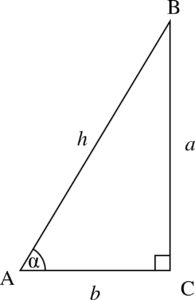# 24 4.0 Introduction – Trigonometry

If a vector is acting in two-dimensions, it can be broken down into a horizontal component and a vertical component. You’ll soon realize that a resultant vector broken down into its components (x and y) resembles a right-angle triangle. To be successful in adding and subtracting vectors, you should be comfortable calculating the components of a right-angle triangle. A right-angle triangle, is a triangle that has one 90 degree angle.We can use the following two concepts to solve the length of the triangle’s side and the angle at the corners:

1. Pythagorean theorem: The length of the hypothenuse (the longest side of the triangle) squared, it equal to the the sum of both sides squared. Using the figure above, we have the equation:

h 2 = a2 + b2

2. Trigonometric ratios: We will use three of the trigonometric ratios: sin, cos and tan. These are ratios because they are expressed in terms of the length of the sides of a right-angled triangle for a specific angle. Let’s take for example, the angle ‘alpha’ in the figure above. The hypothenuse is ‘h’, the side opposite to the angle is ‘a’ and the side adjacent to the angle is ‘b’.

The sin of alpha is equal to the length of the opposite side divided by the length of the hypothenuse:

sin α = a/h

The cos of alpha is equal to the length of the adjacent side divided by the length of the hypothenuse:

cos α = b/h

The tan of alpha is equal to the length of the opposite side divided by the length of the adjacent:

tan α = a/b

A common mnemonic for remembering these relationships is SohCahToa, formed from the first letters of “Sine is opposite over hypotenuse, Cosine is adjacent over hypotenuse, Tangent is opposite over adjacent.”